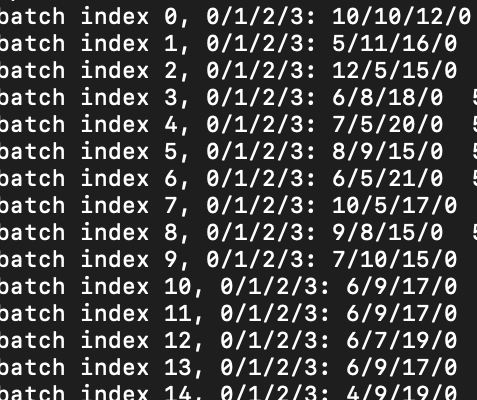# WeightedRandomSampler sampler not working properly

I am using the Weighted random sampler function of PyTorch to sample my classes equally, But while checking the samples of each class in a batch, it seems to sample randomly.

here is a snippet of my code.

def cal_sample_weight(files):
print("file length ",len(files))
labels = [int(f[-5])-1 for f in files]
class_count = [labels.count© for c in np.unique(labels)]
print("class count is ",class_count)
# weights_class = [(1.0/len(np.unique(labels)))/(cc) for cc in class_count]
weights_class = [1.0/(cc) for cc in class_count]
print("Weight class is ", weights_class)
weight_array = np.array([weights_class[l] for l in labels])
print(weight_array.shape)

np.random.seed(0)
random.shuffle(Train_files)
random.shuffle(Val_files)

print([(int(image_path[-5])-1) for image_path in Train_files[0:32]])
Train_labels = [(int(image_path[-5])-1) for image_path in Train_files]
Val_labels = [(int(image_path[-5])-1) for image_path in Val_files]

Train_sample_weight = cal_sample_weight(Train_files)
Val_sample_weight = cal_sample_weight(Val_files)

training_set = Dataset(list_IDs=Train_files,labels=Train_labels, sample_weight=Train_sample_weight, shuffle=True, augment=True)
print("length of train is ", len(training_set))

sampler_train = torch.utils.data.sampler.WeightedRandomSampler(weights = Train_sample_weight, num_samples=len(training_set))

I can’t find any obvious errors in the code.
Could you post the current class distribution you are seeing in each batch?

I assume `Train_labels` contains the labels for the complete `training_set`?
If so you don’t need to wrap it into a `Subset`.

PS: You can post code snippets by wrapping them into three backticks ```, which would make debugging easier.This is the distribution most of the times. Distribution is pretty random. If I remove the subset part and the labels part, then none of the class has samples as 0 in the batch, but the sampling contains majority from class 2,3 and minority from 1,4 which should not be the case.
Its sampling is random. When I run the code, sometimes it samples really badly, sometimes its better. Why is this so?
Yes train labels contains labels for complete set.

``````class Dataset(data.Dataset):
def __init__(self, list_IDs, labels, sample_weight, augment=True, shuffle=True):
#'Initialization'
self.list_IDs = list_IDs
self.labels=labels
self.augment = augment
self.shuffle = shuffle
self.sample_weight = sample_weight
# self.on_epoch_end()
#
def __getitem__(self, index):
np.random.seed(index)
# image_path = np.random.choice(a=self.list_IDs)
image_path = self.list_IDs[index]
my_path=image_path.replace("/home/ken.chang@ccds.io/mnt","/data" )
if self.augment:
image = self.__preprocess_input(image)
label = (int(image_path[-5])-1)
image = TF.to_tensor(image).float()
label = torch.from_numpy(np.array(label))
return image, label

def __len__(self):
return int(len(self.list_IDs))

def on_epoch_end(self):
print("hello_epoch")
if self.shuffle == True:
c = list(zip(self.list_IDs,self.sample_weight, self.labels))
random.shuffle(c)
self.list_IDs, self.sample_weight, self.labels = zip(*c)

def __preprocess_input(self, image):
if np.random.rand(1)>.5:
image = np.fliplr(image)
if np.random.rand(1)>.5:
image = np.flipud(image)
image = rotate(image, angle=np.random.rand(1)*45, mode='nearest', reshape=False)
return image

#draw from each sample with equal class probability
def cal_sample_weight(files):
print("file length ",len(files))
labels = [int(f[-5])-1 for f in files]
class_count = [labels.count(c) for c in np.unique(labels)]
print("class count is ",class_count)
weights_class = [((1.0/len(np.unique(labels)))/(cc)) for cc in class_count]
# weights_class = [1.0/(cc) for cc in class_count]
print("Weight class is ", weights_class)
weight_array = np.array([weights_class[l] for l in labels])
# return weight_array

os.chdir('/data/2015P002510/MammoDensity/scripts_preprocessing/splits')
with open("Train_images_DMIST2.txt", "rb") as fp:
with open("Train_images_DMIST3.txt", "rb") as fp:
with open("Train_images_DMIST4.txt", "rb") as fp:
with open("Train_images_MGH.txt", "rb") as fp:
Train_files = Train_files1+Train_files2+Train_files3+Train_files4
#
with open("Val_images_DMIST2.txt", "rb") as fp:
with open("Val_images_DMIST3.txt", "rb") as fp:
with open("Val_images_DMIST4.txt", "rb") as fp:
with open("Val_images_MGH.txt", "rb") as fp:
Val_files = Val_files1+Val_files2+Val_files3+Val_files4

print([(int(image_path[-5])-1) for image_path in Train_files[0:32]])

random.shuffle(Train_files)
random.shuffle(Val_files)

print([(int(image_path[-5])-1) for image_path in Train_files[0:32]])
Train_labels = [(int(image_path[-5])-1) for image_path in Train_files]
Val_labels = [(int(image_path[-5])-1) for image_path in Val_files]

#
Train_sample_weight = cal_sample_weight(Train_files)
print(Train_sample_weight[0:32])
Val_sample_weight = cal_sample_weight(Val_files)

training_set = Dataset(list_IDs=Train_files,labels=Train_labels, sample_weight=Train_sample_weight, shuffle=True, augment=True)
print("length of train is ", len(training_set))

sampler_train = torch.utils.data.sampler.WeightedRandomSampler(weights = Train_sample_weight, num_samples=len(training_set))

test_set = Dataset(Val_files,labels=Val_labels, sample_weight=Val_sample_weight, augment=False)
print("lenght of val is ", len(test_set))
sampler_test = torch.utils.data.sampler.WeightedRandomSampler(weights = Val_sample_weight, num_samples=len(test_set))

Could you provide some information about your current data distribution, i.e. how many instances do you have from each class?

Hey, I figured this out. Seems that even though I am zipping my weights with the samples while shuffling at the epoch end, the weights with the sampler don’t get shuffled. The weighted random sampler just allocated the probabilities to all the samples once and has no effect of my custom shuffling.

My accuracies are still 1-2% off as compared to my Keras code and I think the reason is ‘no-shuffle’ after each epoch. If I set shuffle=True in the data loader, it throws the error that sampler and shuffle are mutually exclusive options.
Is there a way to shuffle properly with a weighted random sampler?

The weights are used to sample the sample, which already samples “randomly” using the weights as probabilities.
You cannot shuffle the dataset again.

Sorry, what is the meaning of “Randomly” here. Also, does the random seed being used in the WeightedRandomSampler to choose the batch getting changed after each epoch? since otherwise it will be choosing same batch again and again

Random sampling is done using some kind of probability distribution.
If you are shuffling the data, it could be seen as sampling from a uniform distribution without replacement.
Since you are providing the weights to sample each data sample, how should shuffling work in this case?

Inside the `WeightedRandomSampler` this line of code will return the samples.
If the order of the weights and samples is shuffled it won’t make a difference, as `torch.multinomial` will still use the same weight for the corresponding sample.